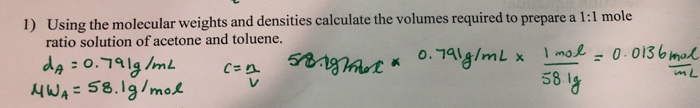# Question Solved1 Answer1) Using the molecular weights and densities calculate the volumes required to prepare a 1:1 mole ratio solution of acetone and toluene. da =0.791g/mL MWA =58.19/mol 51gmot * O.791g/mL x Imol = 0. 013 6 mol C=n 58 g %3DTranscribed Image Text: 1) Using the molecular weights and densities calculate the volumes required to prepare a 1:1 mole ratio solution of acetone and toluene. da =0.791g/mL MWA =58.19/mol 51gmot * O.791g/mL x Imol = 0. 013 6 mol C=n 58 g %3D
More
Transcribed Image Text: 1) Using the molecular weights and densities calculate the volumes required to prepare a 1:1 mole ratio solution of acetone and toluene. da =0.791g/mL MWA =58.19/mol 51gmot * O.791g/mL x Imol = 0. 013 6 mol C=n 58 g %3D